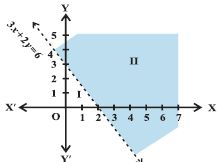Email us to get an instant 20% discount on highly effective K-12 Math & English kwizNET Programs!

#### Online Quiz (WorksheetABCD)

Questions Per Quiz = 2 4 6 8 10

### High School Mathematics - 27.8 Graphical Representation of Solutions to Inequalities

 Example: Solve 3x+2y> 6 graphically. Solution: The dotted line is the graph of the given inequality.This dotted line divides the xy plane in two half planes I and II. We select a point say (0,0) which lies in one of the half planes. We determine if this point satisfies the give inequality 3(0) + 2(0) > 6 which is false. The shaded half plane II excluding the points on the line is the solution region of the inequality. Directions: Draw the graphs.
 Q 1: Solve 3x-6>=0 graphically.Answer: Q 2: Solve 3x+y <= 12 graphically.Answer: Q 3: Solve 2x+y >=6 graphically.Answer: Q 4: Solve 3x-6>=0 graphically.Answer: Q 5: Solve y+8>=2x graphically.Answer: Q 6: Solve x-y<=2 graphically.Answer: Q 7: Solve y<2 graphically.Answer: Q 8: Solve 3x-6>=0 graphically.Answer: Question 9: This question is available to subscribers only! Question 10: This question is available to subscribers only!

#### Subscription to kwizNET Learning System offers the following benefits:

• Unrestricted access to grade appropriate lessons, quizzes, & printable worksheets
• Instant scoring of online quizzes
• Progress tracking and award certificates to keep your student motivated
• Unlimited practice with auto-generated 'WIZ MATH' quizzes